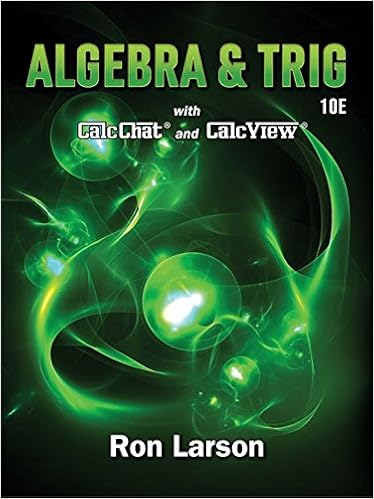Use the Zero Product Property - Raevyn Davis Use the Zero...

• Homework Help
• 3
• 100% (31) 31 out of 31 people found this document helpful

This preview shows page 1 - 2 out of 3 pages.

We have textbook solutions for you!
The document you are viewing contains questions related to this textbook.The document you are viewing contains questions related to this textbook.
Chapter P / Exercise 97
Algebra & Trigonometry
LarsonExpert Verified
Raevyn Davis 4/2/2017 Use the Zero Product Property 1. A child launches a toy rocket from the top of a slide at the park. Suppose the equation 16t^2 + 28t + 8 = 0 can be used to find how many seconds it will take for the rocket to hit the ground. a. Write the equation in factored form i. 4(t – 2)(4t + 1) b. Use the zero-product property to solve the equation. Show all the steps needed to find both answers. c. Explain how the solution relates to this situation.
2. A manufacturing company manufactures a cardboard box
with a square base and a height of 15 inches. Suppose the equation x^2 + 60x – 7,200 = 0 can be used to find the length and width of the base of the box, each measuring x inches. a. Write the equation in factored form.
We have textbook solutions for you!
The document you are viewing contains questions related to this textbook.The document you are viewing contains questions related to this textbook.
Chapter P / Exercise 97
Algebra & Trigonometry
LarsonExpert Verified
•••